# Using DHARMa to check Bayesian models fitted with brms

UPDATE 26 October 2022: There is now a DHARMa.helpers package that facilitates checking Bayesian `brms` models with DHARMa. Check it out!

The R package DHARMa is incredibly useful to check many different kinds of statistical models. It can be used with Bayesian models too, although it requires a few more lines of code.

Here I develop an example using DHARMa to check a Bayesian hierarchical generalised linear model fitted with the also fantastic brms package.

## Example dataset

First, let’s simulate some count data with a hierarchical structure (random effect) and overdispersion:

``library(DHARMa)``
``````testdata <- createData(sampleSize = 1000,
intercept = 0,
fixedEffects = 1,
numGroups = 20,
family = poisson(),
overdispersion = 0.5)

``````##   ID observedResponse Environment1 group time         x         y
## 1  1                1  -0.68065204     1    1 0.2875775 0.2736227
## 2  2                0  -0.71096830     1    2 0.7883051 0.5938669
## 3  3                1  -0.70163922     1    3 0.4089769 0.1601848
## 4  4                1   0.02886852     1    4 0.8830174 0.8534302
## 5  5                0  -0.01434539     1    5 0.9404673 0.8477392
## 6  6                2   0.23268554     1    6 0.0455565 0.4778868``````

We have simulated counts (`observedResponse`) in 20 sites as a function of an environmental variable (`Environment1`):

``````library(ggplot2)

ggplot(testdata) +
geom_point(aes(Environment1, observedResponse)) +
facet_wrap(~group) ``````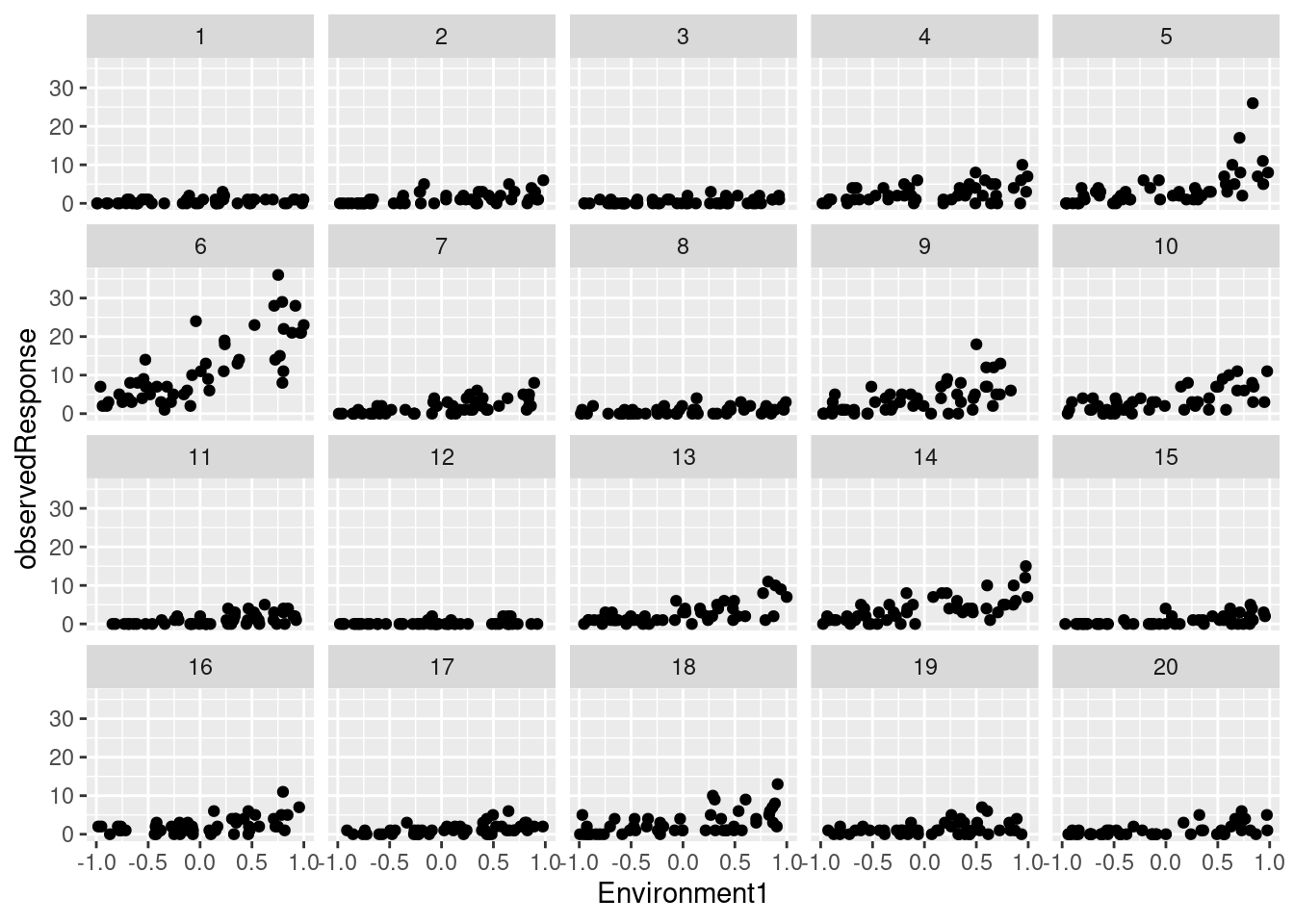## Fitting the model with brms

First, we try a non-hierarchical Poisson GLM of observed counts as a function of environment. For simplicity, using default priors here.

``library(brms)``
``````model <- brm(observedResponse ~ Environment1,
data = testdata,
family = poisson(),
cores = 4)``````
``summary(model)``
``````##  Family: poisson
## Formula: observedResponse ~ Environment1
##    Data: testdata (Number of observations: 1000)
##   Draws: 4 chains, each with iter = 2000; warmup = 1000; thin = 1;
##          total post-warmup draws = 4000
##
## Population-Level Effects:
##              Estimate Est.Error l-95% CI u-95% CI Rhat Bulk_ESS Tail_ESS
## Intercept        0.69      0.03     0.64     0.74 1.00     1597     1974
## Environment1     1.05      0.04     0.97     1.14 1.00     1707     2047
##
## Draws were sampled using sampling(NUTS). For each parameter, Bulk_ESS
## and Tail_ESS are effective sample size measures, and Rhat is the potential
## scale reduction factor on split chains (at convergence, Rhat = 1).``````

## Model checking with DHARMa

To use DHARMa with `brms` (and other Bayesian packages), we must use the `createDHARMa` function, which takes the posterior simulations and the observed response to generate a DHARMa object, as `simulateResiduals` do with other supported model types.

``````model.check <- createDHARMa(
simulatedResponse = t(posterior_predict(model)),
observedResponse = testdata\$observedResponse,
fittedPredictedResponse = apply(t(posterior_epred(model)), 1, mean),
integerResponse = TRUE)

plot(model.check)``````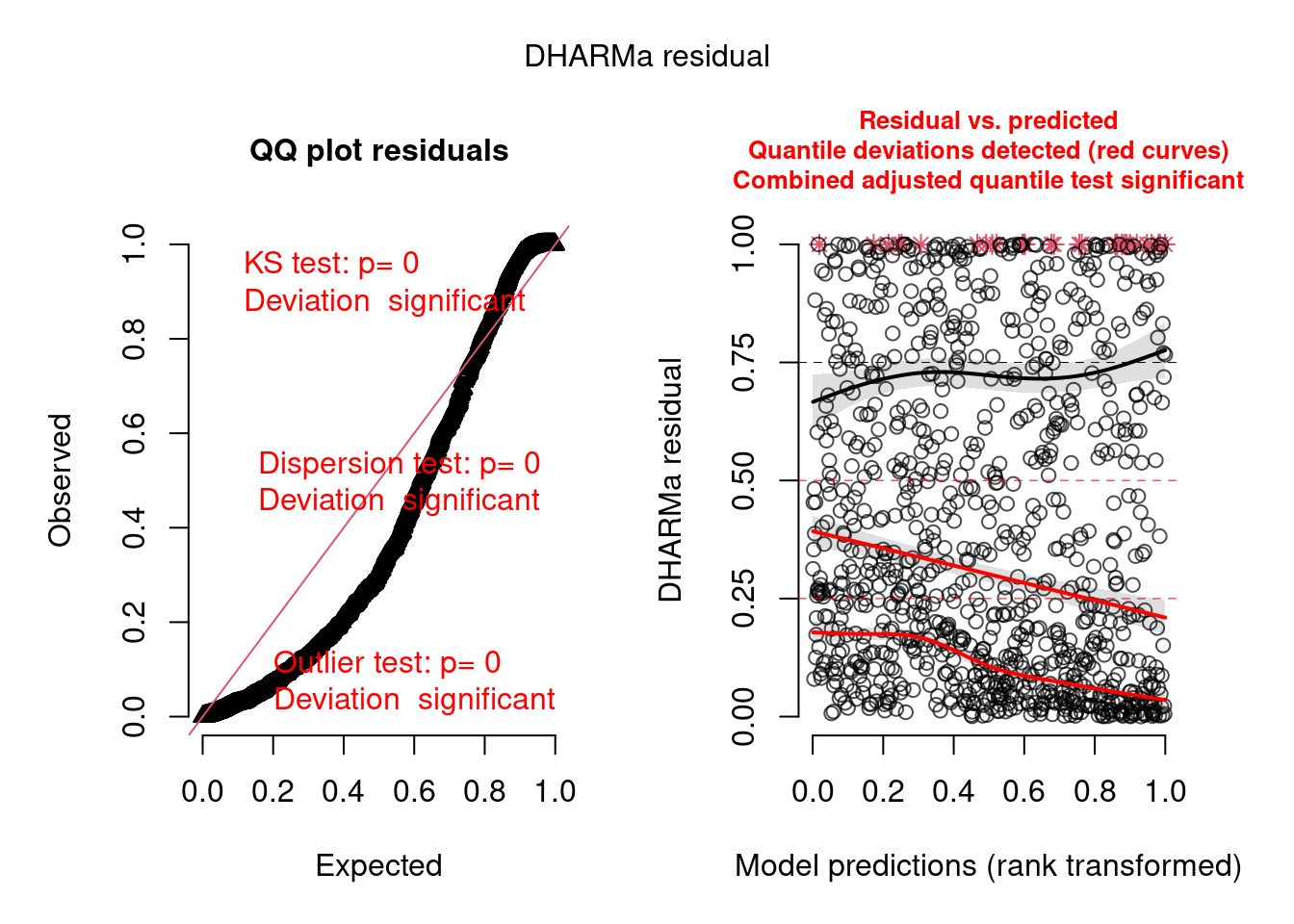We can see there are problems with this model, which we will try to fix below. The posterior predictive check from `bayesplot` confirms the model is not adequate:

``pp_check(model, nsamples = 100) + xlim(0, 20)``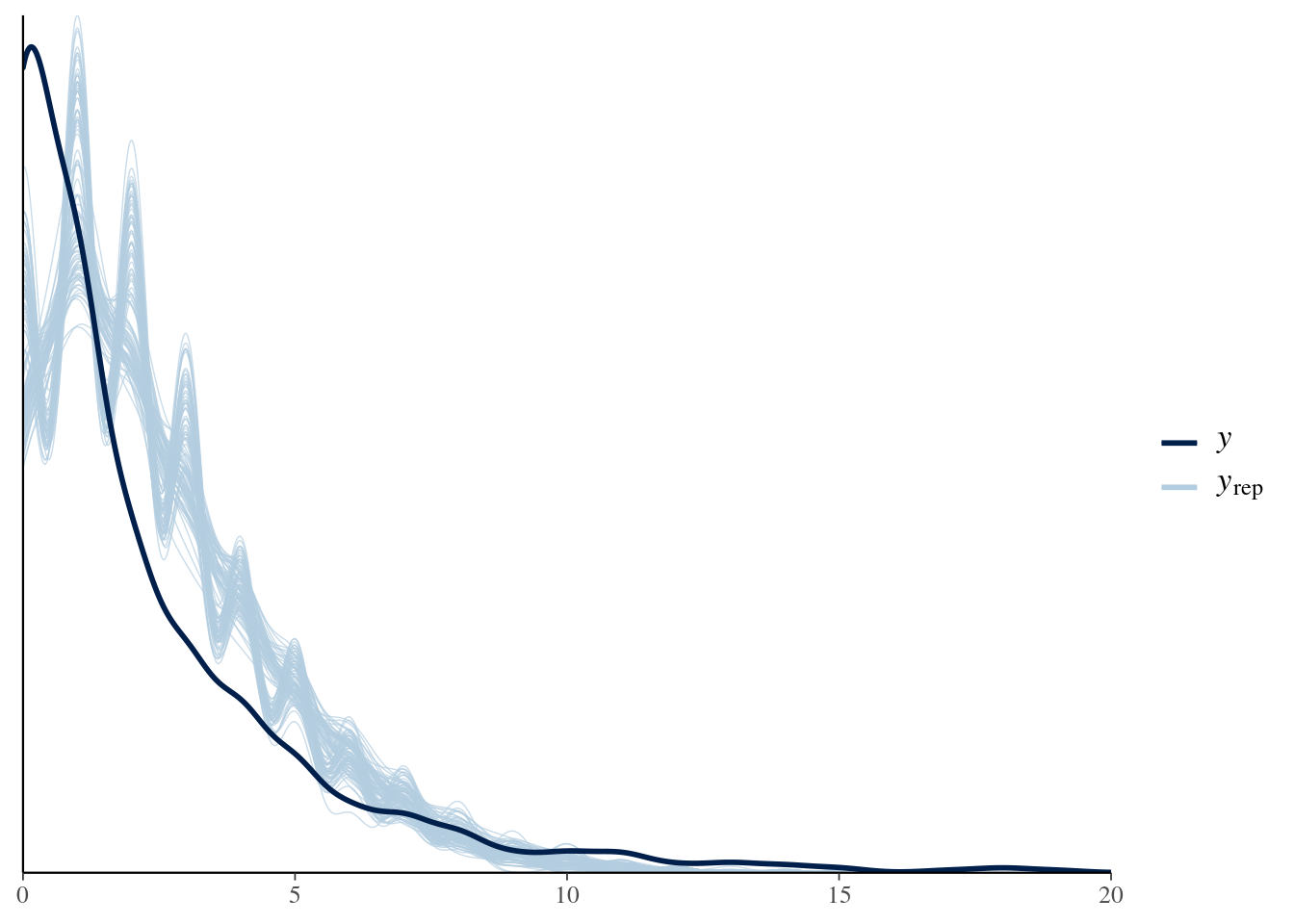## Automating DHARMa checks for brms models

To simplify the code, let’s write a function to automate the creation and examination of DHARMa objects from fitted brms models:

``````check_brms <- function(model,             # brms model
integer = FALSE,   # integer response? (TRUE/FALSE)
plot = TRUE,       # make plot?
...                # further arguments for DHARMa::plotResiduals
) {

mdata <- brms::standata(model)
if (!"Y" %in% names(mdata))
stop("Cannot extract the required information from this brms model")

dharma.obj <- DHARMa::createDHARMa(
simulatedResponse = t(brms::posterior_predict(model, ndraws = 1000)),
observedResponse = mdata\$Y,
fittedPredictedResponse = apply(
t(brms::posterior_epred(model, ndraws = 1000, re.form = NA)),
1,
mean),
integerResponse = integer)

if (isTRUE(plot)) {
plot(dharma.obj, ...)
}

invisible(dharma.obj)

}``````

Note this function is used here for convenience and will not work with all brms models (e.g. models with more than one response).

## Improving the model

Now, let’s try to fix the model. The DHARMa plot above shows that our model is not accounting for potential differences among groups (sites), and there is likely overdispersion (see DHARMa vignette for detailed explanations of how to interpret these plots).

Let’s start adding a varying intercept (random effect) to account for sites/groups:

``````model2 <- brm(observedResponse ~ Environment1 + (1 | group),
data = testdata,
family = poisson(),
cores = 4,
iter = 4000)``````
``summary(model2)``
``````##  Family: poisson
## Formula: observedResponse ~ Environment1 + (1 | group)
##    Data: testdata (Number of observations: 1000)
##   Draws: 4 chains, each with iter = 4000; warmup = 2000; thin = 1;
##          total post-warmup draws = 8000
##
## Group-Level Effects:
## ~group (Number of levels: 20)
##               Estimate Est.Error l-95% CI u-95% CI Rhat Bulk_ESS Tail_ESS
## sd(Intercept)     0.95      0.18     0.68     1.37 1.00      877     1944
##
## Population-Level Effects:
##              Estimate Est.Error l-95% CI u-95% CI Rhat Bulk_ESS Tail_ESS
## Intercept        0.33      0.21    -0.10     0.75 1.01      781     1209
## Environment1     1.05      0.04     0.97     1.12 1.00     3923     4460
##
## Draws were sampled using sampling(NUTS). For each parameter, Bulk_ESS
## and Tail_ESS are effective sample size measures, and Rhat is the potential
## scale reduction factor on split chains (at convergence, Rhat = 1).``````

Check with DHARMa using the function we just created:

``model2.check <- check_brms(model2, integer = TRUE)``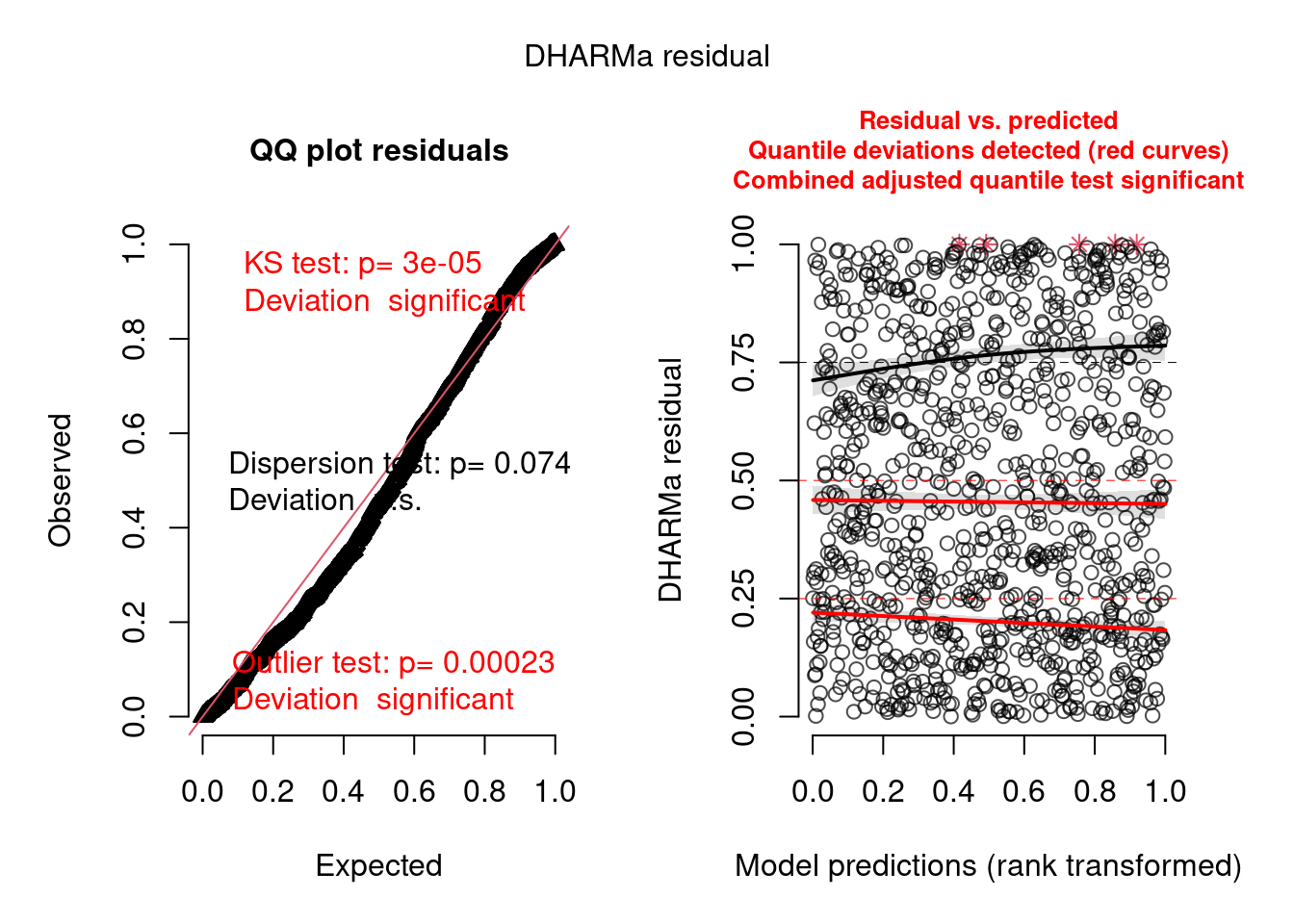There are still problems, but the residuals show a better pattern now. They are now quite homogeneous among sites:

``plot(model2.check, form = testdata\$group)``But there are still problems. One of them seems to be overdispersion:

``testDispersion(model2.check)``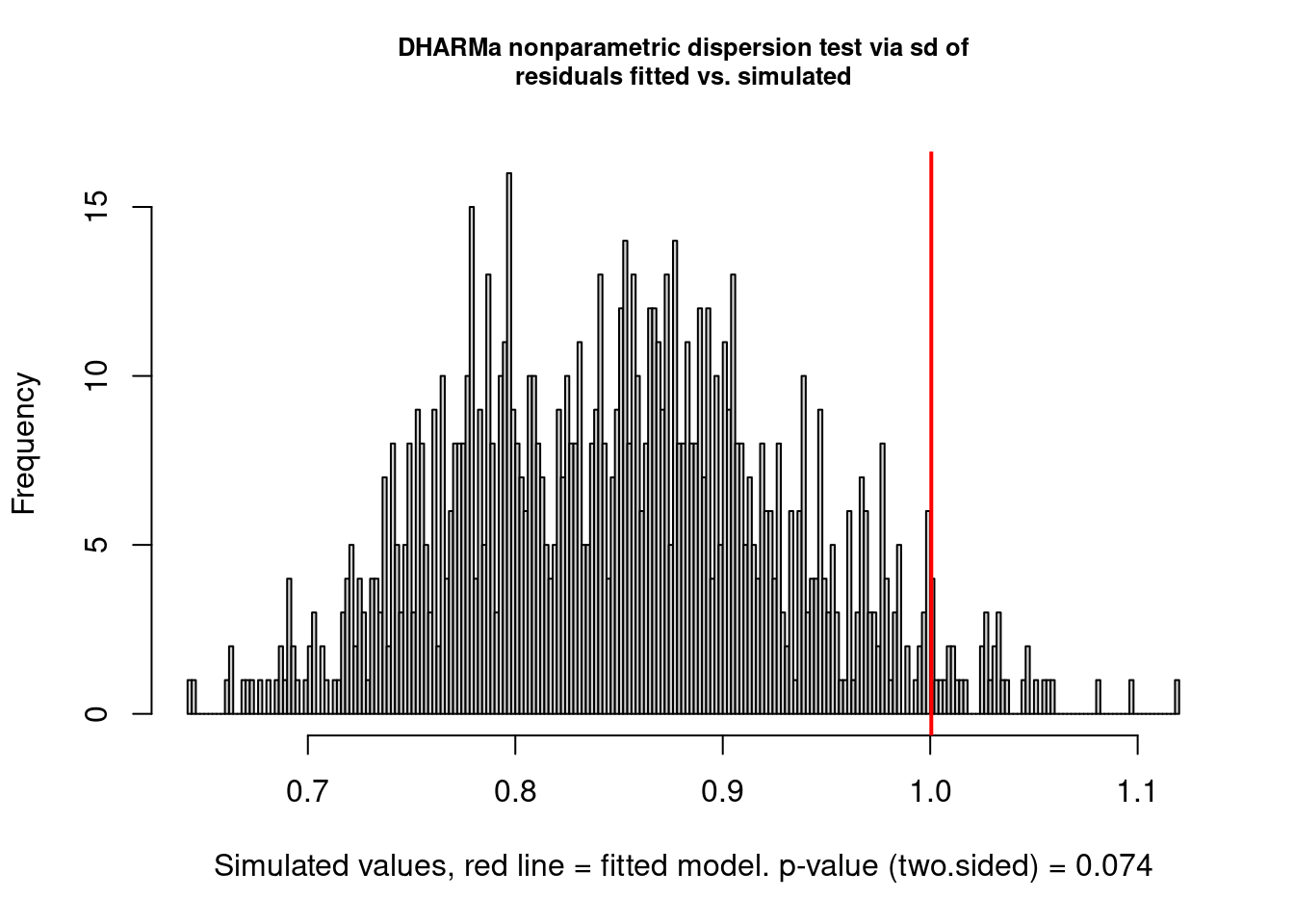``````##
##  DHARMa nonparametric dispersion test via sd of residuals fitted vs.
##  simulated
##
## data:  simulationOutput
## dispersion = 1.1758, p-value = 0.074
## alternative hypothesis: two.sided``````

## Improving the model further

Let’s add an observation-level random effect to try to account for overdispersion:

``````testdata\$obs.id <- 1:nrow(testdata)

model3 <- brm(observedResponse ~ Environment1 + (1 | group) + (1 | obs.id),
data = testdata,
family = poisson(),
cores = 4,
iter = 4000)``````
``summary(model3)``
``````##  Family: poisson
## Formula: observedResponse ~ Environment1 + (1 | group) + (1 | obs.id)
##    Data: testdata (Number of observations: 1000)
##   Draws: 4 chains, each with iter = 4000; warmup = 2000; thin = 1;
##          total post-warmup draws = 8000
##
## Group-Level Effects:
## ~group (Number of levels: 20)
##               Estimate Est.Error l-95% CI u-95% CI Rhat Bulk_ESS Tail_ESS
## sd(Intercept)     0.97      0.18     0.69     1.39 1.00     1552     2831
##
## ~obs.id (Number of levels: 1000)
##               Estimate Est.Error l-95% CI u-95% CI Rhat Bulk_ESS Tail_ESS
## sd(Intercept)     0.45      0.04     0.38     0.52 1.00     3113     4371
##
## Population-Level Effects:
##              Estimate Est.Error l-95% CI u-95% CI Rhat Bulk_ESS Tail_ESS
## Intercept        0.22      0.21    -0.20     0.63 1.00      854     1776
## Environment1     1.07      0.05     0.97     1.18 1.00     6430     6334
##
## Draws were sampled using sampling(NUTS). For each parameter, Bulk_ESS
## and Tail_ESS are effective sample size measures, and Rhat is the potential
## scale reduction factor on split chains (at convergence, Rhat = 1).``````

Check:

``model3.check <- check_brms(model3, integer = TRUE)``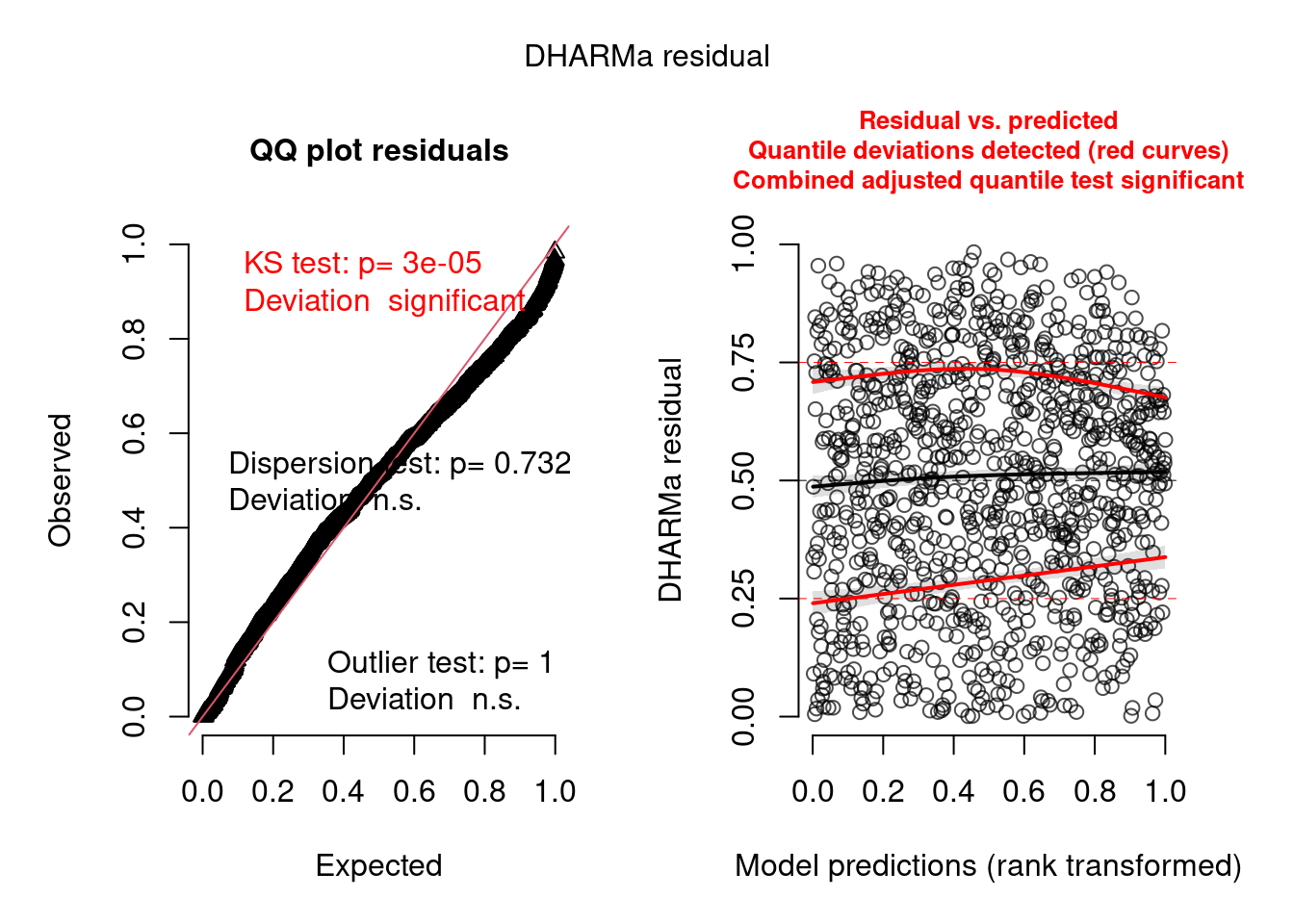There are still some warnings, although the observation-level random effect seems to have corrected for overdispersion:

``testDispersion(model3.check)````````##
##  DHARMa nonparametric dispersion test via sd of residuals fitted vs.
##  simulated
##
## data:  simulationOutput
## dispersion = 0.96409, p-value = 0.732
## alternative hypothesis: two.sided``````

## Improve the model even further

Let’s try using a negative binomial distribution rather than a Poisson:

``````model4 <- brm(observedResponse ~ Environment1 + (1 | group),
data = testdata,
family = negbinomial(),
cores = 4,
iter = 4000)

summary(model4)``````
``````##  Family: negbinomial
##   Links: mu = log; shape = identity
## Formula: observedResponse ~ Environment1 + (1 | group)
##    Data: testdata (Number of observations: 1000)
##   Draws: 4 chains, each with iter = 4000; warmup = 2000; thin = 1;
##          total post-warmup draws = 8000
##
## Group-Level Effects:
## ~group (Number of levels: 20)
##               Estimate Est.Error l-95% CI u-95% CI Rhat Bulk_ESS Tail_ESS
## sd(Intercept)     0.95      0.17     0.69     1.37 1.00     1098     1744
##
## Population-Level Effects:
##              Estimate Est.Error l-95% CI u-95% CI Rhat Bulk_ESS Tail_ESS
## Intercept        0.31      0.22    -0.12     0.74 1.00      885     1613
## Environment1     1.07      0.05     0.97     1.17 1.00     4924     4697
##
## Family Specific Parameters:
##       Estimate Est.Error l-95% CI u-95% CI Rhat Bulk_ESS Tail_ESS
## shape     4.81      0.75     3.57     6.45 1.00     5178     4762
##
## Draws were sampled using sampling(NUTS). For each parameter, Bulk_ESS
## and Tail_ESS are effective sample size measures, and Rhat is the potential
## scale reduction factor on split chains (at convergence, Rhat = 1).``````

Check:

``model4.check <- check_brms(model4, integer = TRUE)``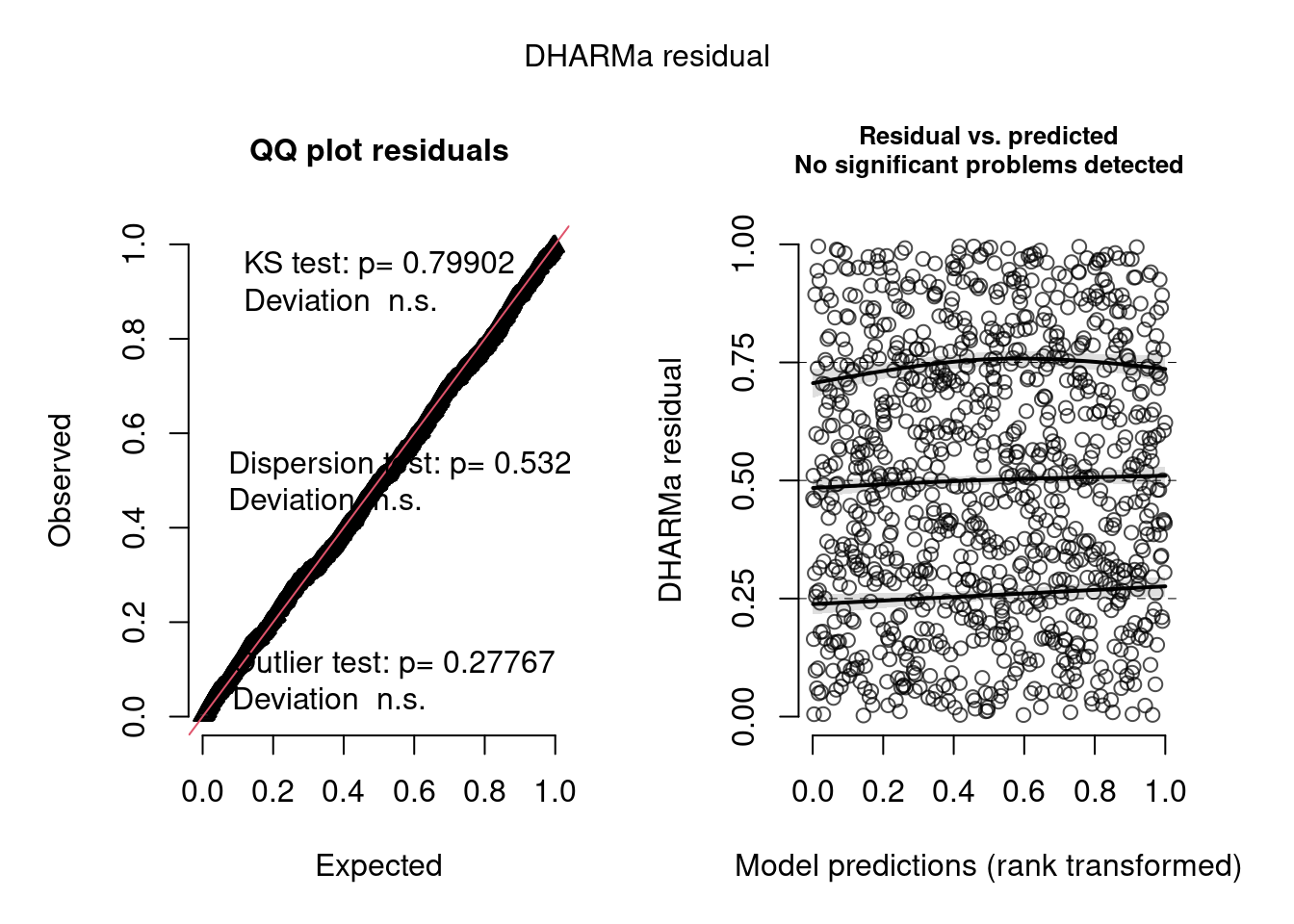``plot(model4.check, form = testdata\$Environment1)``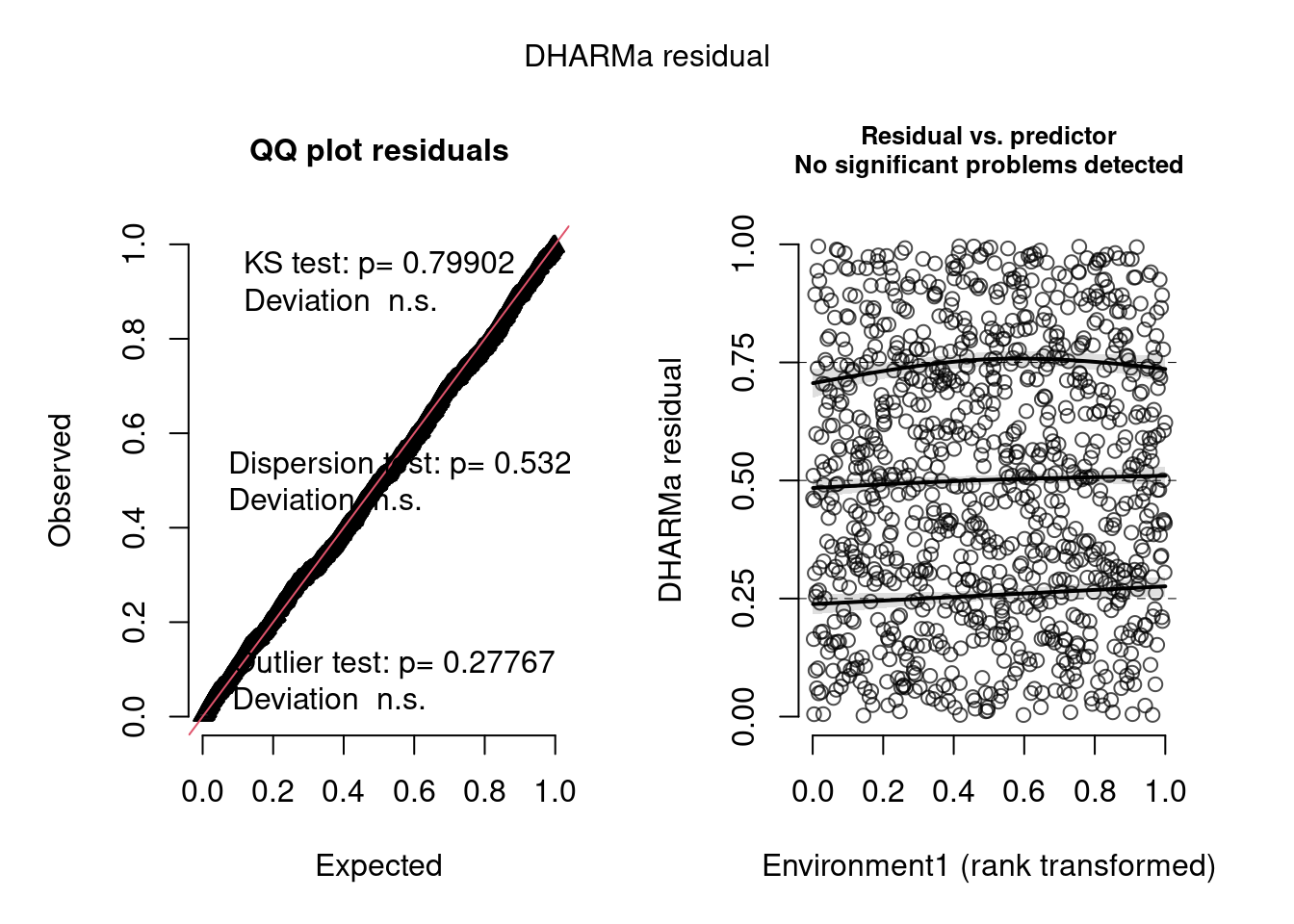``plot(model4.check, form = testdata\$group)``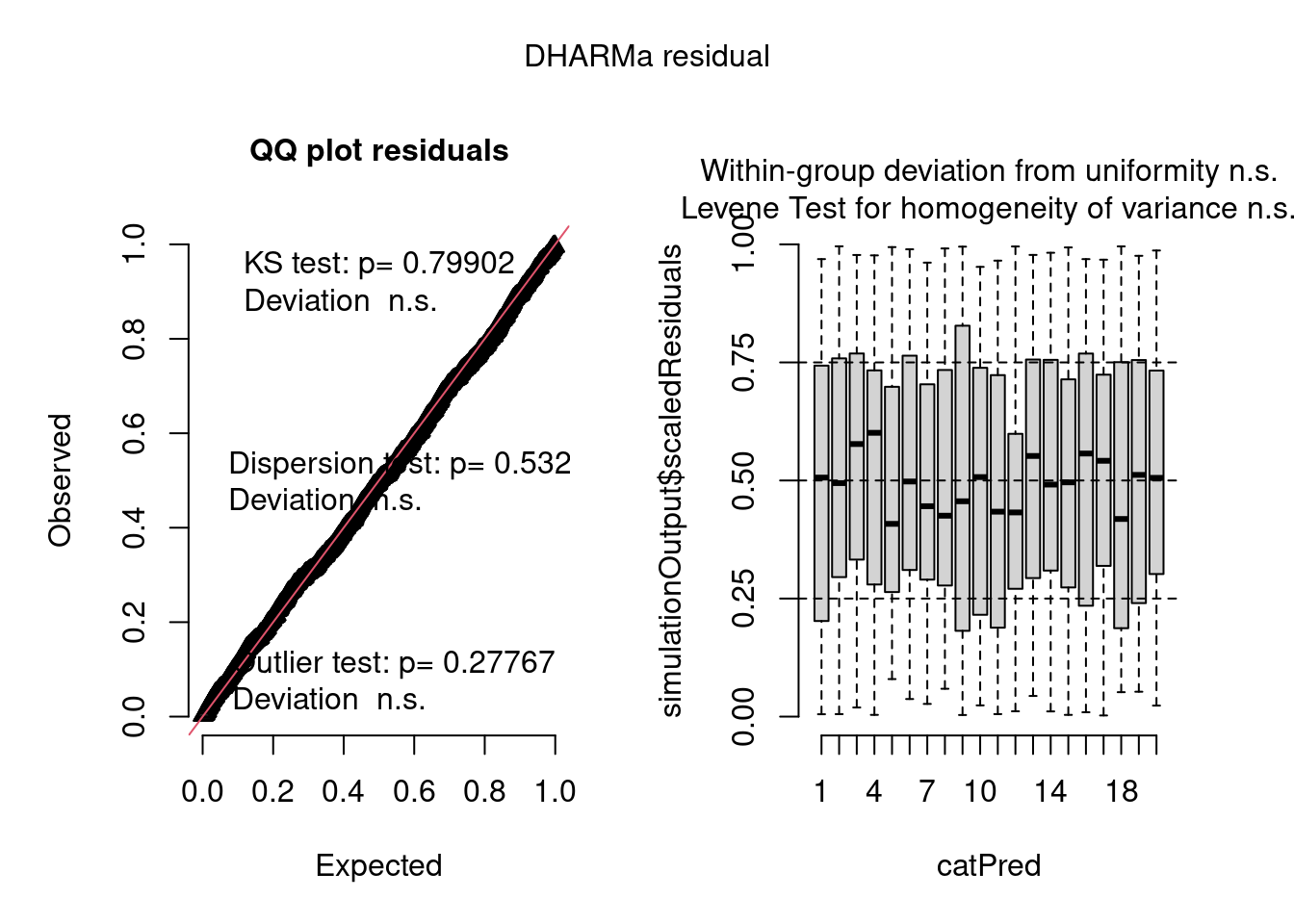The negative binomial model looks better in this case.

Let’s check it with `bayesplot` too:

``pp_check(model4, nsamples = 100) + xlim(0, 20)``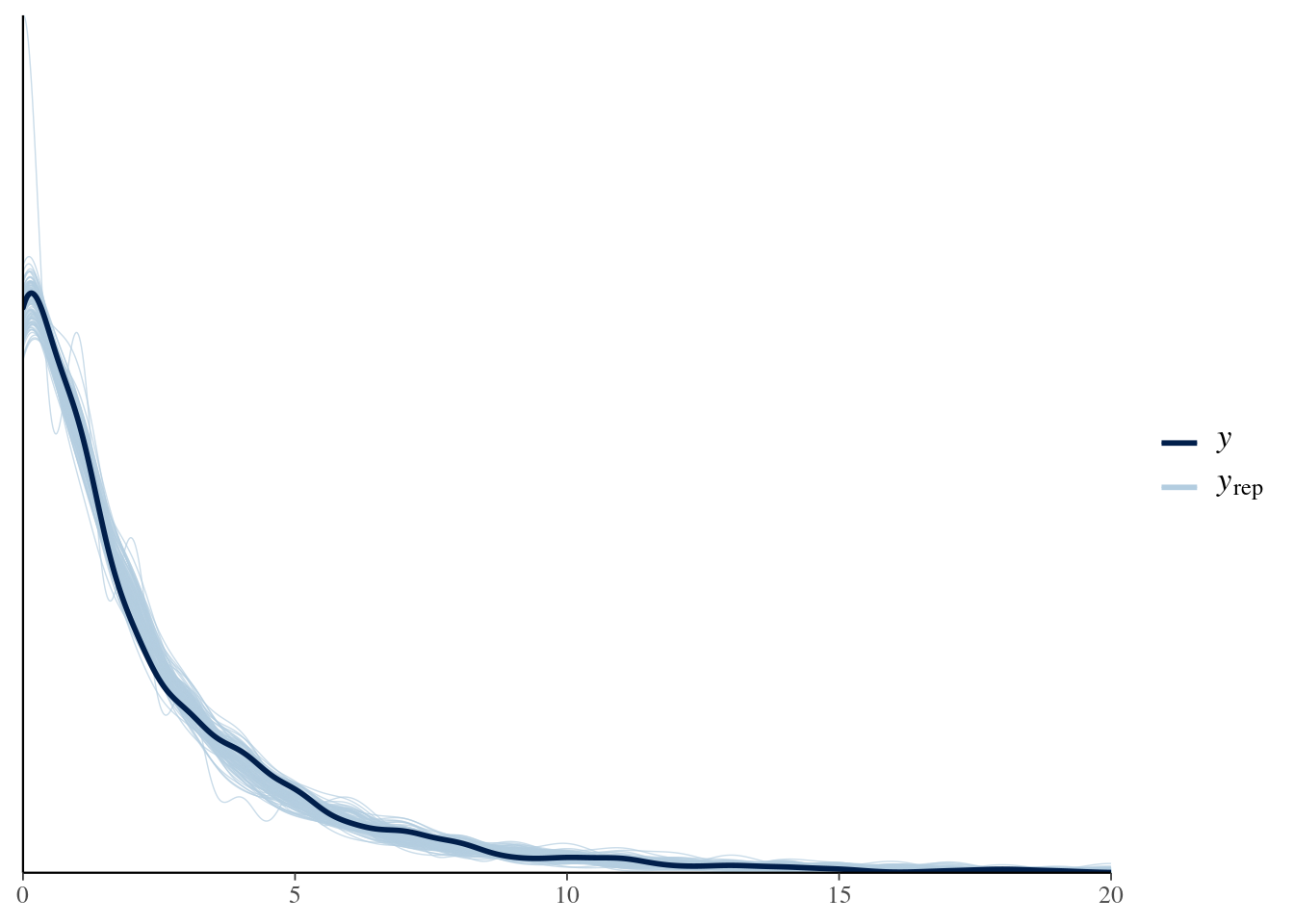``````library(bayesplot)

ppc_rootogram(y = testdata\$observedResponse,
yrep = posterior_predict(model4, nsamples = 1000)) +
xlim(0, 20)``````## Conclusion

DHARMa can greatly facilitate thorough checking and improvement of Bayesian models, as those fitted with `brms`.

Prof. Florian Hartig kindly revised a draft of this post.##### Francisco Rodríguez-Sánchez
###### Researcher

Computational Ecologist & Data Scientist.